# Electronics and Communication Engineering - Analog Electronics

16.

The output V0 in figure is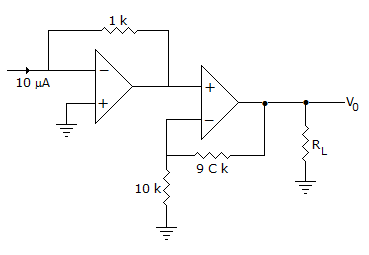A. -100 V B. -100 mV C. 10 V D. 10 mV

Explanation:

Input to non-inverting op-amp is -10 x 10-6 x 103 = -10 mV.

Therefore output =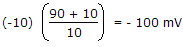= -100 mV.

17.

In a CE amplifier the input impedance is equal to the ratio of

 A. ac base voltage to ac base current B. ac base voltage to ac emitter current C. ac emitter voltage to ac collector current D. ac collector voltage to ac collector current

Explanation:

Input is applied to base with emitter grounded. The input impedance is the ratio of ac base voltage to ac base current.

18.

For a system to work, as oscillator the total phase shift of the loop gain must be equal to

 A. 90° B. 45° C. 270° D. 360°

Explanation:

Gain of system with + ve feedback =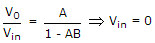for oscillation

but V0 ≠ 0

so, that 1 - AB = 0AB = 1 ∠0° or 360°.

19.

An amplifier has a large ac input signal. The clipping occurs on both the peaks. The output voltage will be nearly a

 A. sine wave B. square wave C. triangular wave D. (a) or (c)

Explanation:

When a sinusoidal voltage is clipped on both sides it resembles a square wave.

20.

The transistor of following figure in Si diode with a base current of 40 μA and ICBO = 0, if VBB = 6V, RE = 2 kΩ and β = 90, IBQ = 20 μA then RB =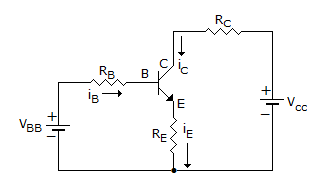A. 200 kΩ B. 265 kΩ C. 150 kΩ D. 100 kΩ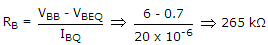.